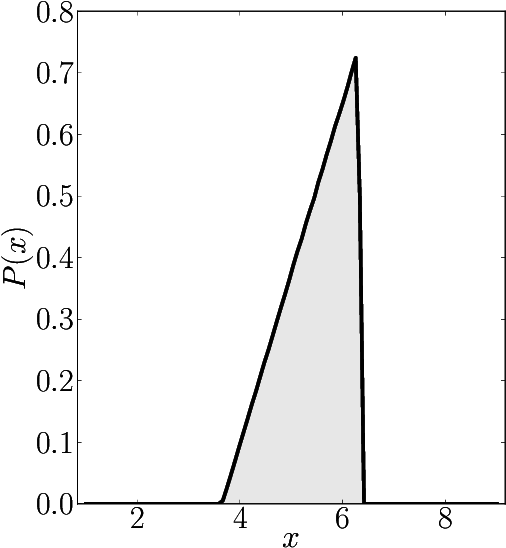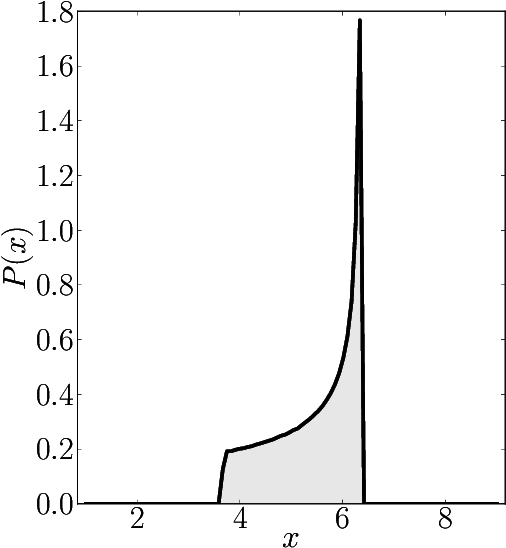# Hermitian matrices

In the case of Hermitian matrices the numerical shadow is a one dimensional distribution.

## Diagonal matrices

### Example 1

The matrix is $\mathrm{diag}(1, 2, 3, 4, 5, 6, 7, 8, 9)$

Numerical shadow with respect to complex product states Numerical shadow with respect to real product states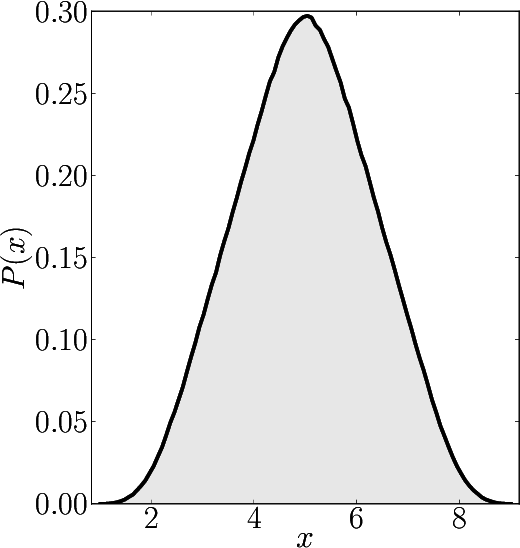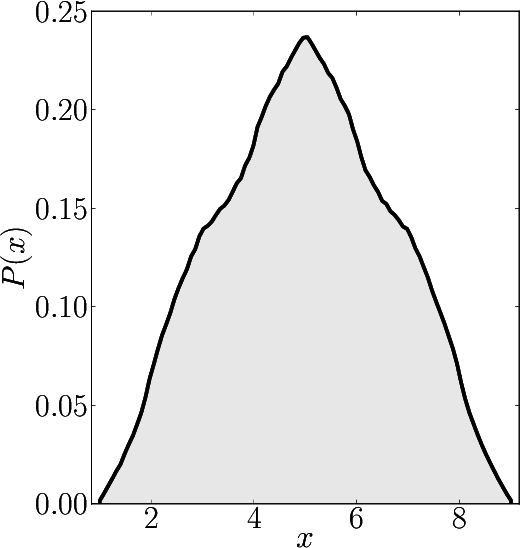Numerical shadow with respect to complex entangled states Numerical shadow with respect to real entangled states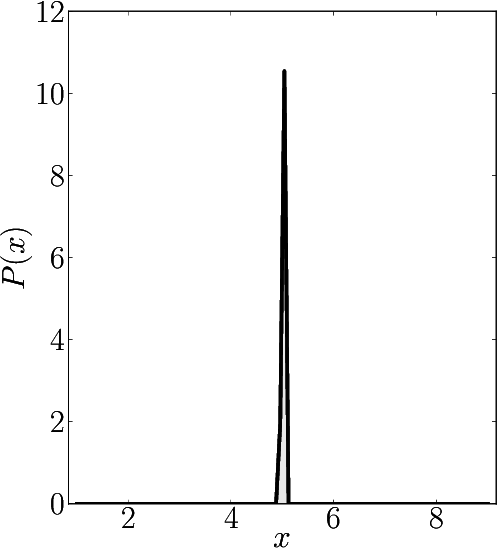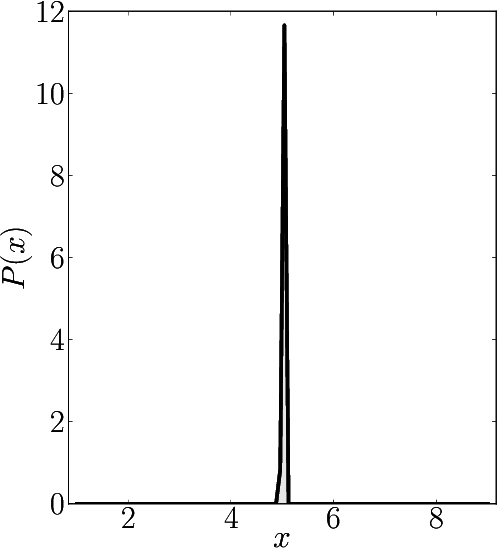### Example 2

The matrix is $\mathrm{diag}(1, 2, 2, 4, 5, 6, 7, 8, 9)$

Numerical shadow with respect to complex product states Numerical shadow with respect to real product states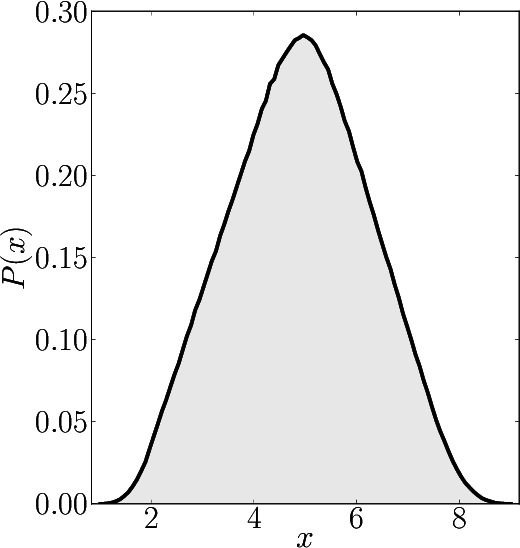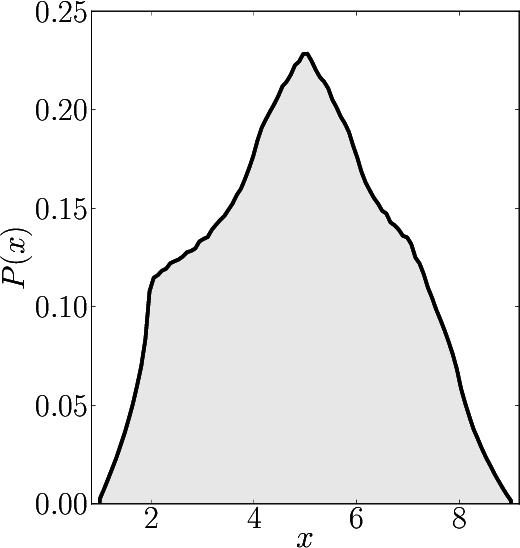Numerical shadow with respect to complex entangled states Numerical shadow with respect to real entangled states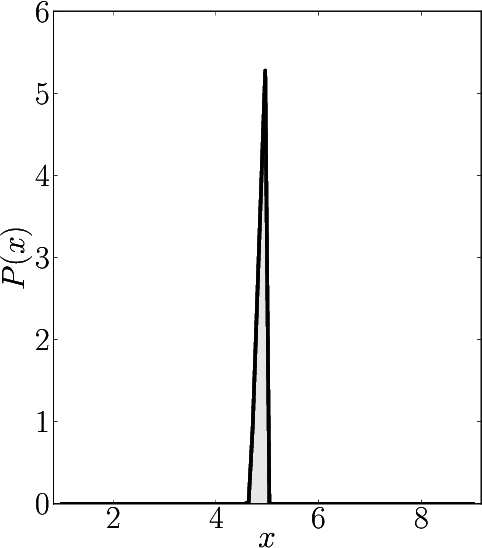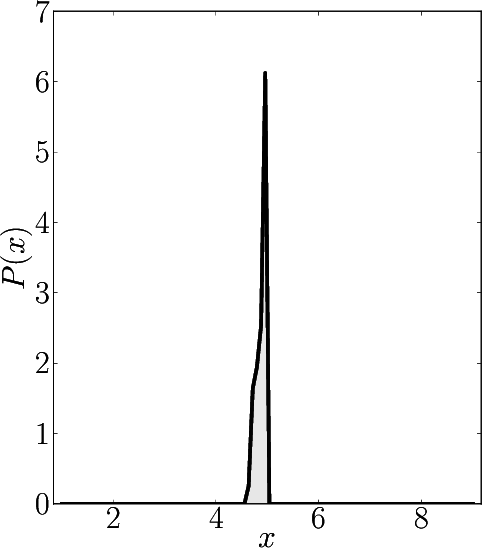### Example 3

The matrix is $\mathrm{diag}(1, 2, 2, 2, 5, 6, 7, 8, 9)$

Numerical shadow with respect to complex product states Numerical shadow with respect to real product states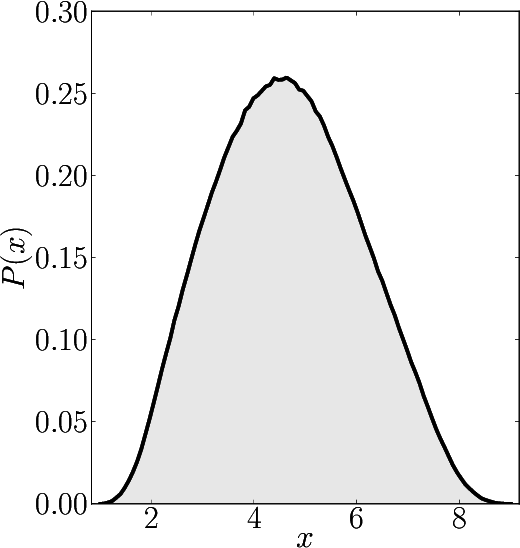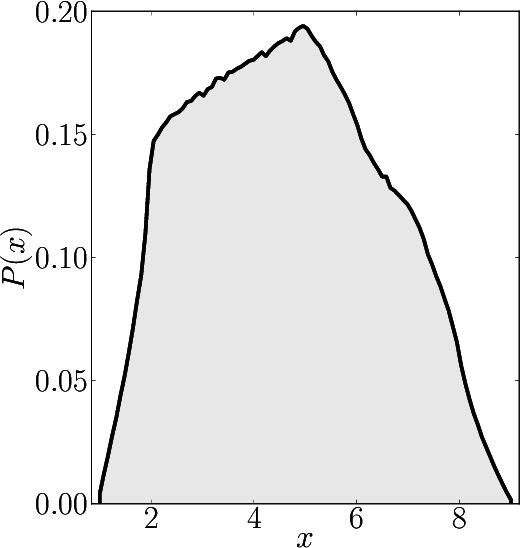Numerical shadow with respect to complex entangled states Numerical shadow with respect to real entangled states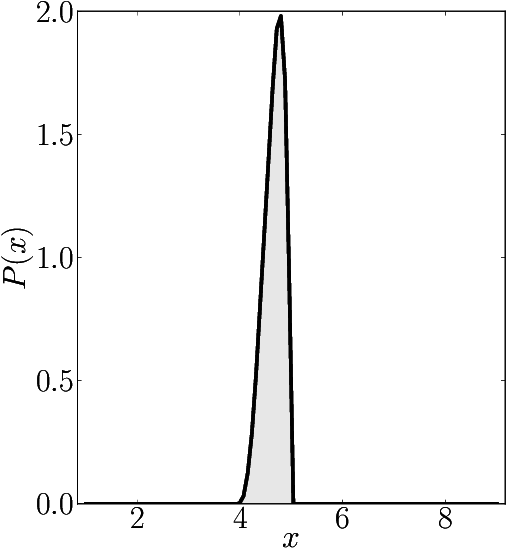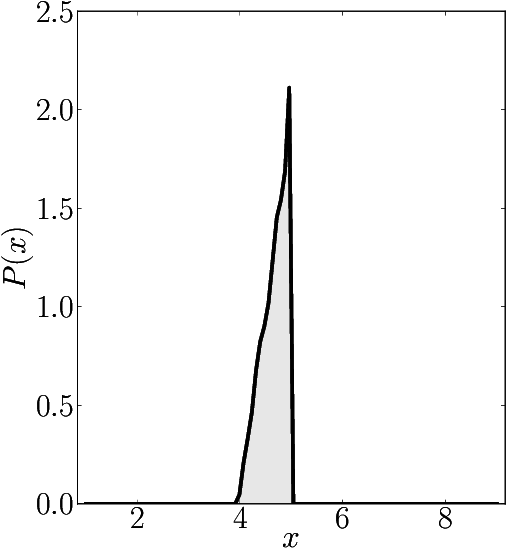### Example 4

The matrix is $\mathrm{diag}(1, 2, 2, 2, 2, 2, 2, 8, 9)$

Numerical shadow with respect to complex product states Numerical shadow with respect to real product states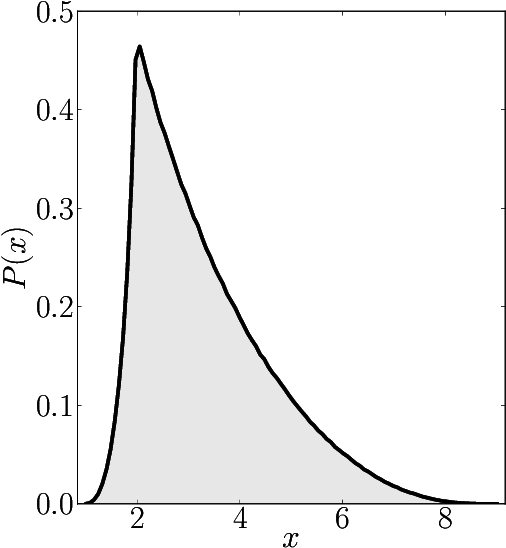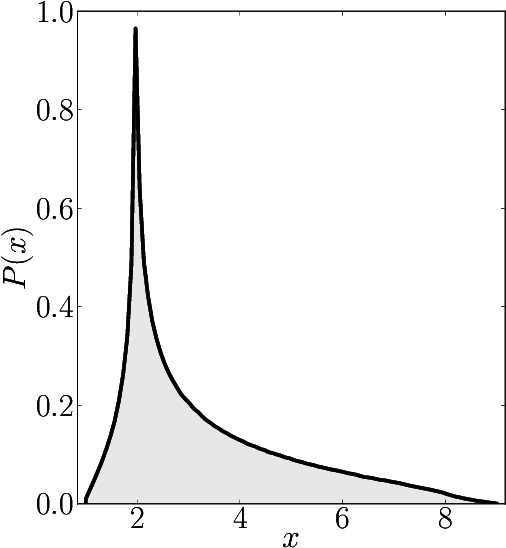Numerical shadow with respect to complex entangled states Numerical shadow with respect to real entangled states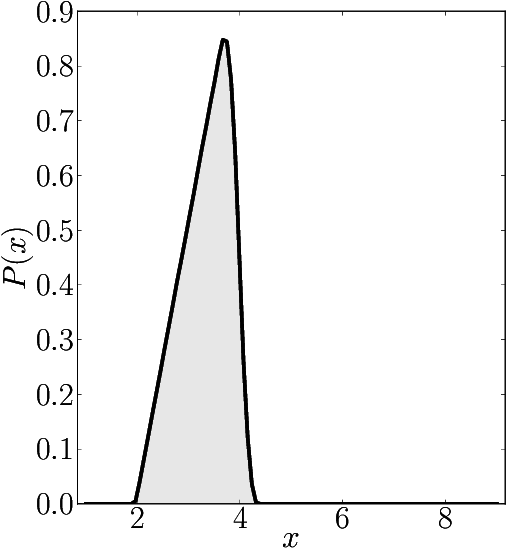### Example 5

The matrix is $\mathrm{diag}(1, 2, 2, 2, 2, 2, 2, 2, 9)$

Numerical shadow with respect to complex product states Numerical shadow with respect to real product states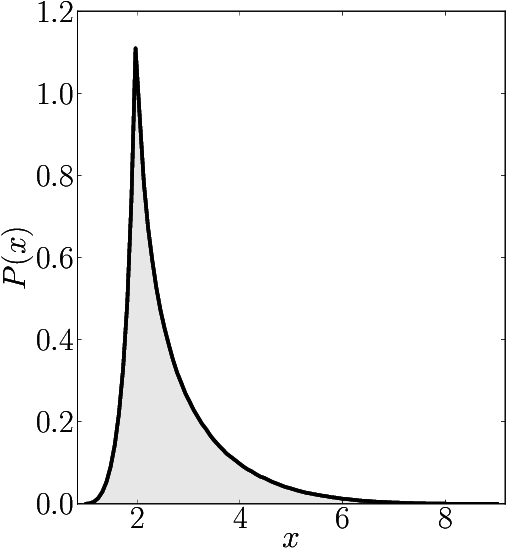Numerical shadow with respect to complex entangled states Numerical shadow with respect to real entangled states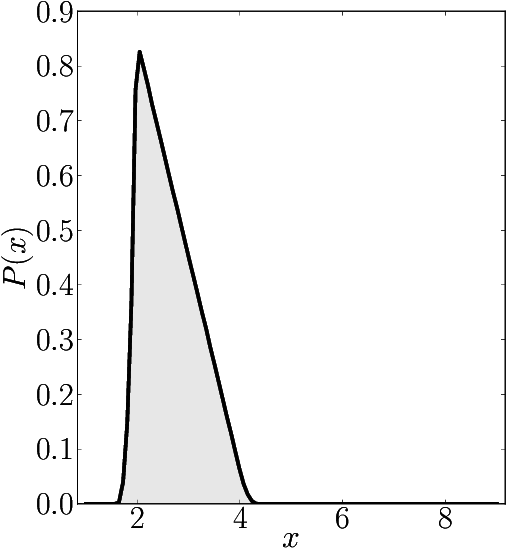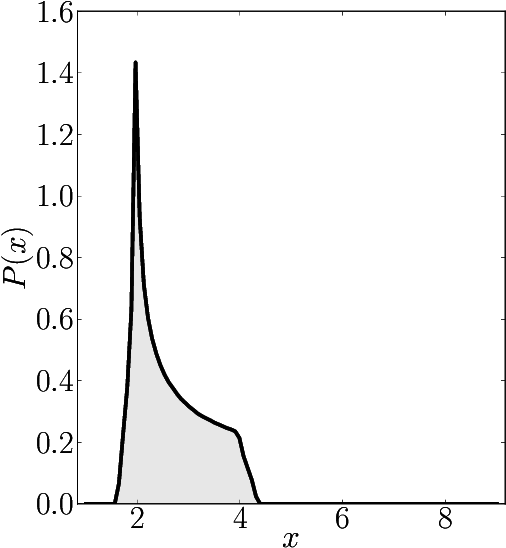### Example 6

The matrix is $\mathrm{diag}(1, 2, 2, 2, 2, 6, 6, 6, 9)$

Numerical shadow with respect to complex product states Numerical shadow with respect to real product states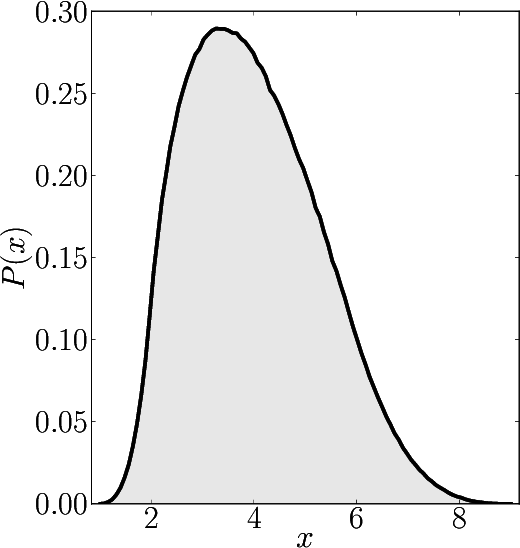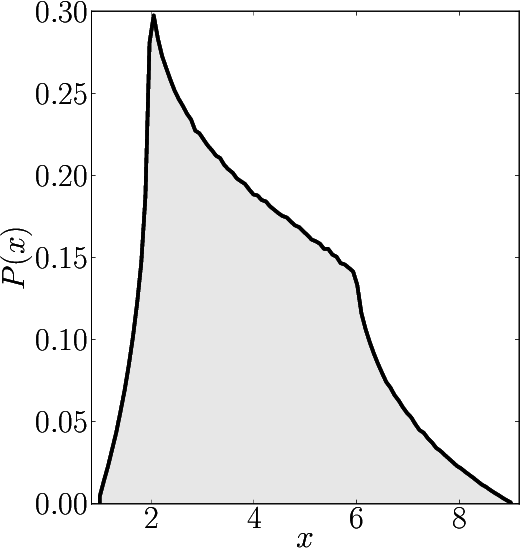Numerical shadow with respect to complex entangled states Numerical shadow with respect to real entangled states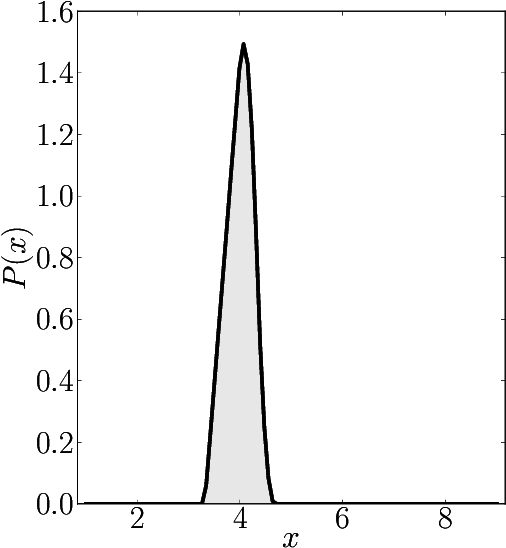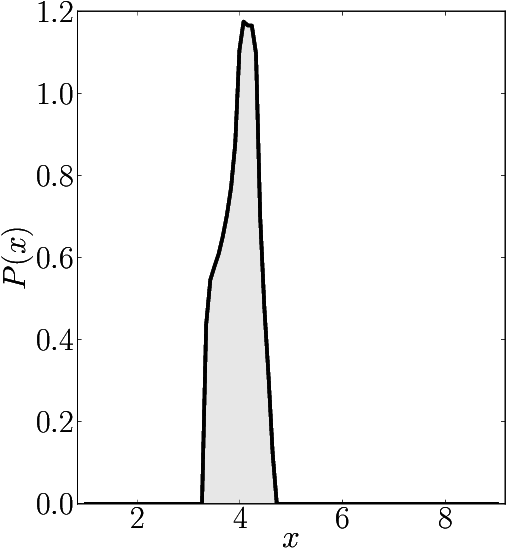### Example 7

The matrix is $\mathrm{diag}(1, 2, 2, 2, 6, 6, 6, 8, 9)$

Numerical shadow with respect to complex product states Numerical shadow with respect to real product states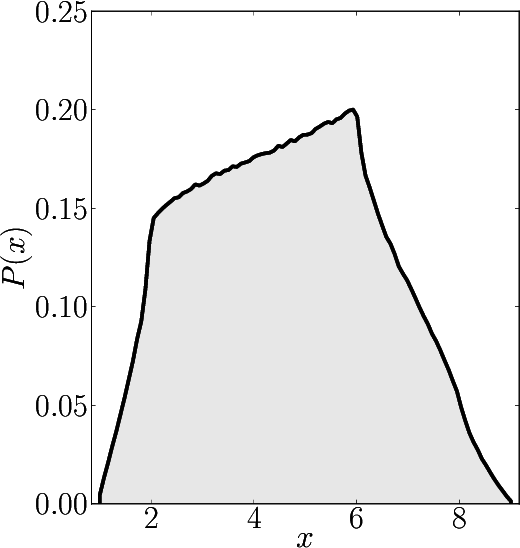Numerical shadow with respect to complex entangled states Numerical shadow with respect to real entangled states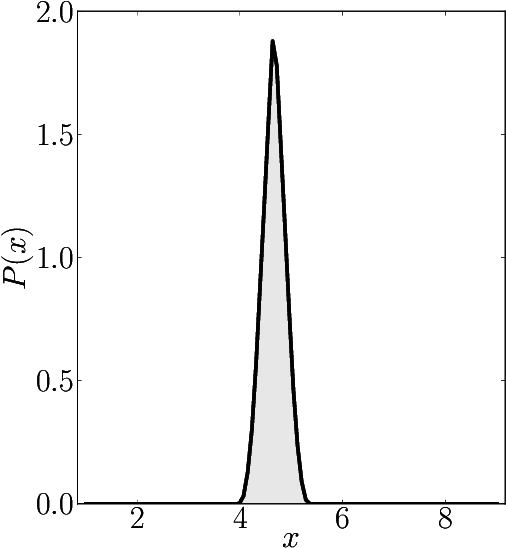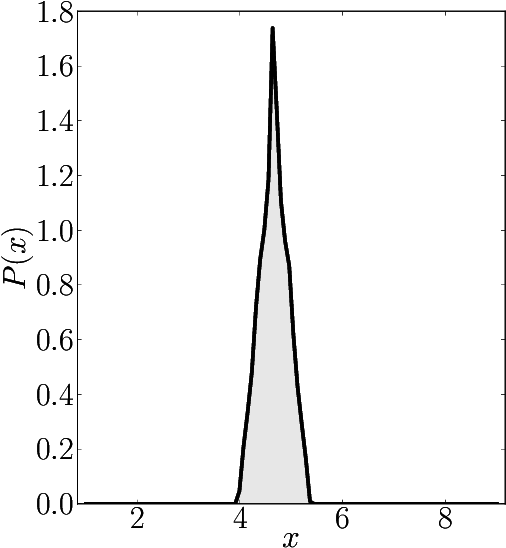### Example 8

The matrix is $\mathrm{diag}(1, 1, 1, 1, 9, 9, 9, 9, 9)$

Numerical shadow with respect to complex product states Numerical shadow with respect to real product states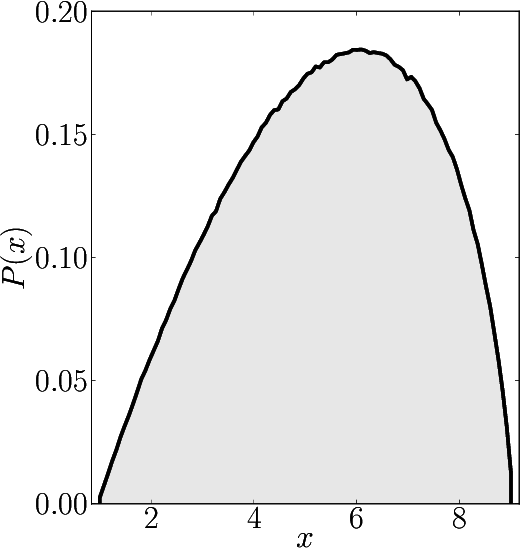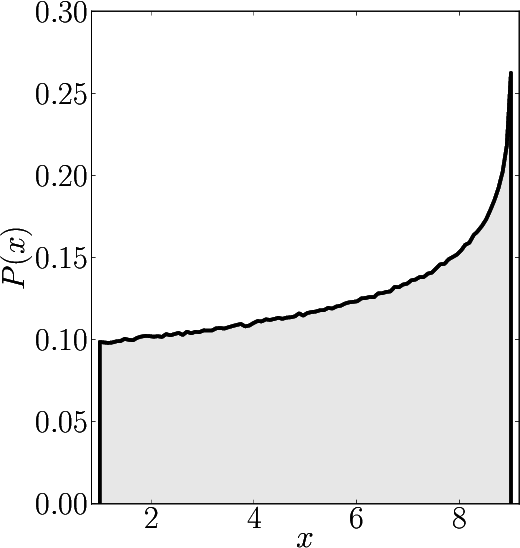Numerical shadow with respect to complex entangled states Numerical shadow with respect to real entangled states